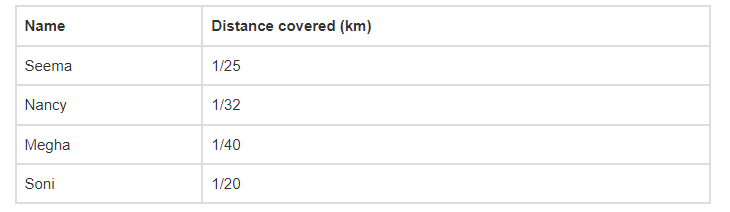# Four friends had a competition to see

Question:

Four friends had a competition to see how far could they hop on one foot. The table given shows the distance covered by each.(a) How farther did Soni hop than Nancy?

(b) What is the total distance covered by Seema and Megha?

(c) Who walked farther, Nancy or Megha?

Solution:

The LCM of the denominators 25, 32, 40 and 20 is 800

∴ 1/25 = [(1×32)/ (25×32)] = (32/800)

(1/32) = [(1×25)/ (32×25)] = (25/800)

(1/40) = [(1×20)/ (40×20)] = (20/800)

(1/20) = [(1×40)/ (20×40)] = (40/800)

Then,

(a) Soni hop more than Nancy = (40/800) – (25/800)

= (40 – 25)/800

= (15/800)

= 3/160 km

(b) The total distance covered by Seema and Megha = (32/800) + (20/800)

= (32 + 20)/800

= (52/800)

= 13/200 km

(c) Nancy walked farther.